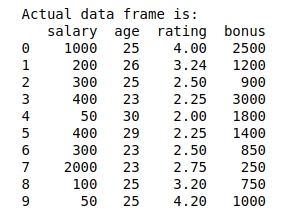• #5, First Floor, 4th Street Dr. Subbarayan Nagar Kodambakkam, Chennai-600 024 Landmark : Samiyar Madam
• pro@slogix.in
• +91- 81240 01111

### How to calculate correlation coefficient for a data set using python?

###### Description

To calculate the correlation coefficient for a data frame in python.

###### Process

Take a sample dataset.

Store it as rows and columns using data frame.

Call the correlation function bydf.corr(method)

By using a value of correlation coefficients,we can find the relationship between the variables.

###### Sample Code

#import library function
import pandas as pd
#sample data
data={‘salary’:[1000,200,300,400,50,400,300,2000,
100,50],
‘age’:[25,26,25,23,30,29,23,23,25,25],
‘rating’:[4,3.24,2.5,2.25,2,2.25,2.5,2.75,3.2,4.2],
‘bonus’:[2500,1200,900,3000,1800,1400,850,250,750,
1000]}
#store in rows and column using pandas data frame
df=pd.DataFrame(data)
print(“Actual data framusing correlation e is:”)
print(df)
#finding the correlation
correlation = df.corr(method=’pearson’)
print(“\n”)
print(“The correlation matrix is:\n”,correlation)

###### Screenshots